Скачать презентацию Conditional Conditional Statements Vocabulary Conditional A compound

a79cfff9f9236c814389961115ca9ff5.ppt

• Количество слайдов: 16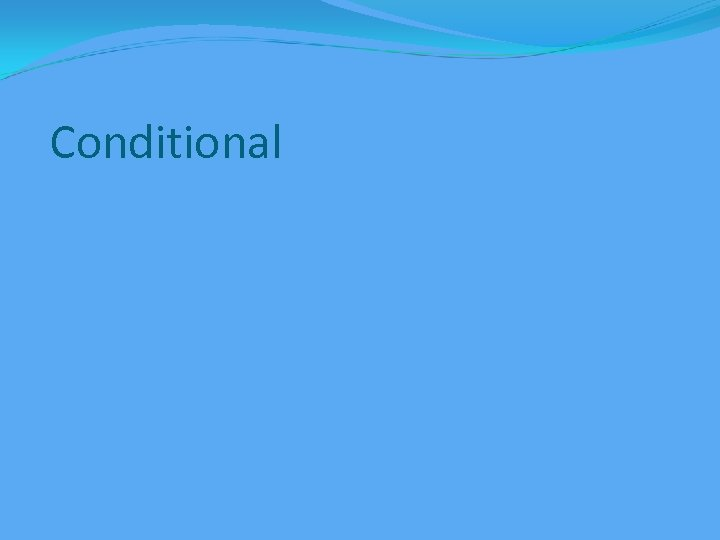Conditional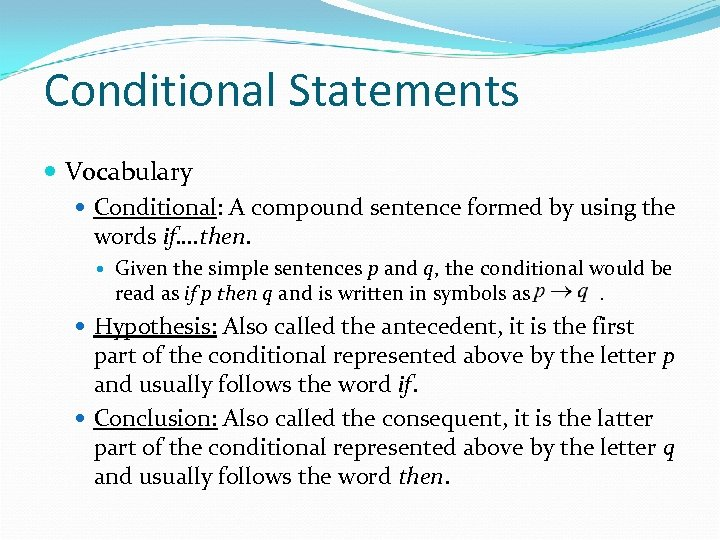Conditional Statements Vocabulary Conditional: A compound sentence formed by using the words if…. then. Given the simple sentences p and q, the conditional would be read as if p then q and is written in symbols as . Hypothesis: Also called the antecedent, it is the first part of the conditional represented above by the letter p and usually follows the word if. Conclusion: Also called the consequent, it is the latter part of the conditional represented above by the letter q and usually follows the word then.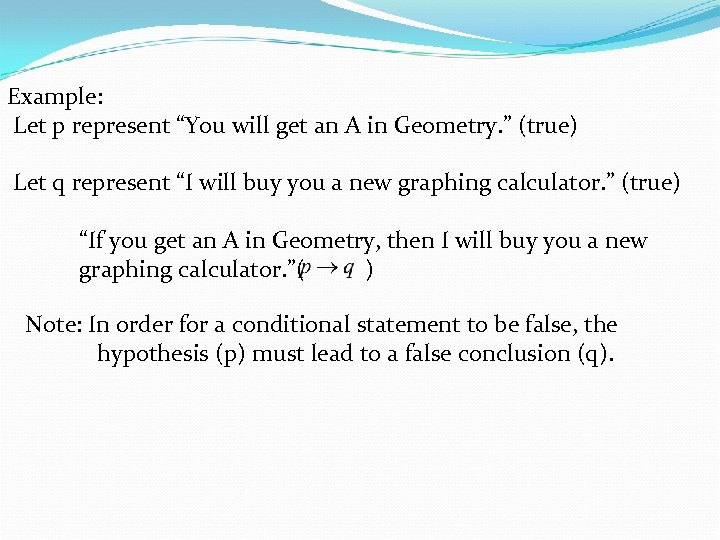Example: Let p represent “You will get an A in Geometry. ” (true) Let q represent “I will buy you a new graphing calculator. ” (true) “If you get an A in Geometry, then I will buy you a new graphing calculator. ”( ) Note: In order for a conditional statement to be false, the hypothesis (p) must lead to a false conclusion (q).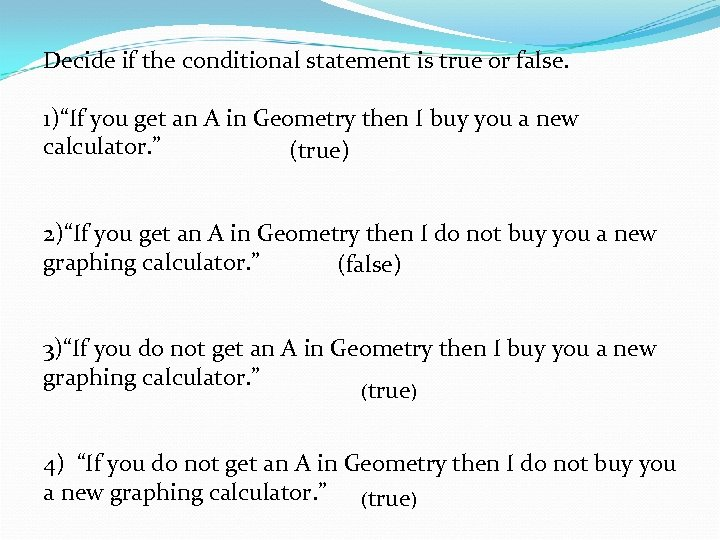Decide if the conditional statement is true or false. 1)“If you get an A in Geometry then I buy you a new calculator. ” (true) 2)“If you get an A in Geometry then I do not buy you a new graphing calculator. ” (false) 3)“If you do not get an A in Geometry then I buy you a new graphing calculator. ” (true) 4) “If you do not get an A in Geometry then I do not buy you a new graphing calculator. ” (true)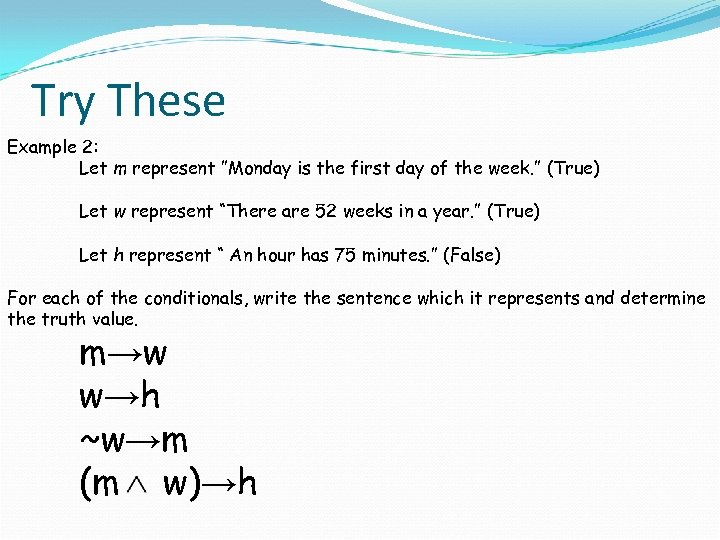Try These Example 2: Let m represent ”Monday is the first day of the week. ” (True) Let w represent “There are 52 weeks in a year. ” (True) Let h represent “ An hour has 75 minutes. ” (False) For each of the conditionals, write the sentence which it represents and determine the truth value. m→w w→h ~w→m (m w)→h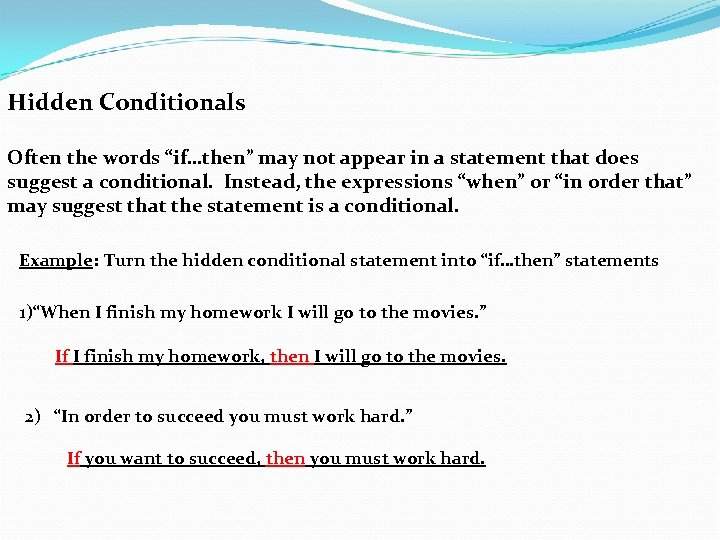Hidden Conditionals Often the words “if…then” may not appear in a statement that does suggest a conditional. Instead, the expressions “when” or “in order that” may suggest that the statement is a conditional. Example: Turn the hidden conditional statement into “if…then” statements 1)“When I finish my homework I will go to the movies. ” If I finish my homework, then I will go to the movies. 2) “In order to succeed you must work hard. ” If you want to succeed, then you must work hard.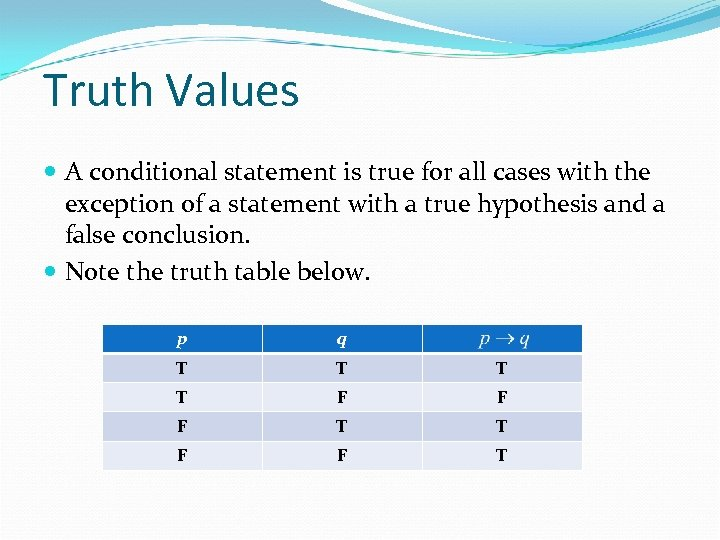Truth Values A conditional statement is true for all cases with the exception of a statement with a true hypothesis and a false conclusion. Note the truth table below. p q T T F F F T T F F T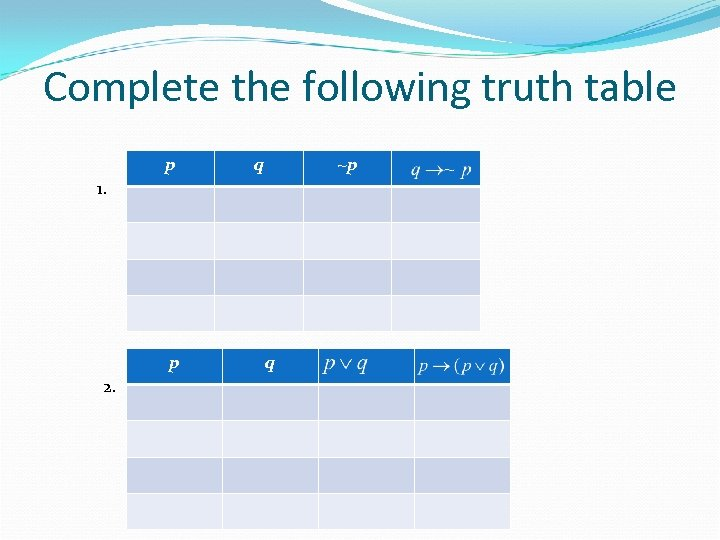Complete the following truth table p q ~p 1. p 2. q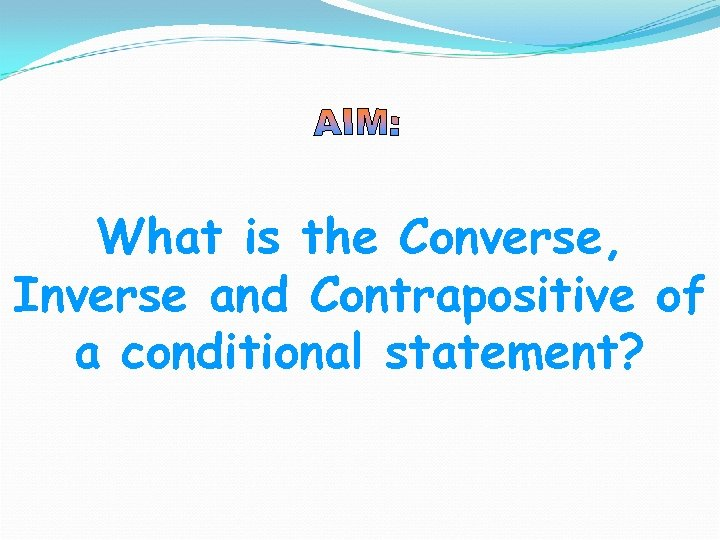What is the Converse, Inverse and Contrapositive of a conditional statement?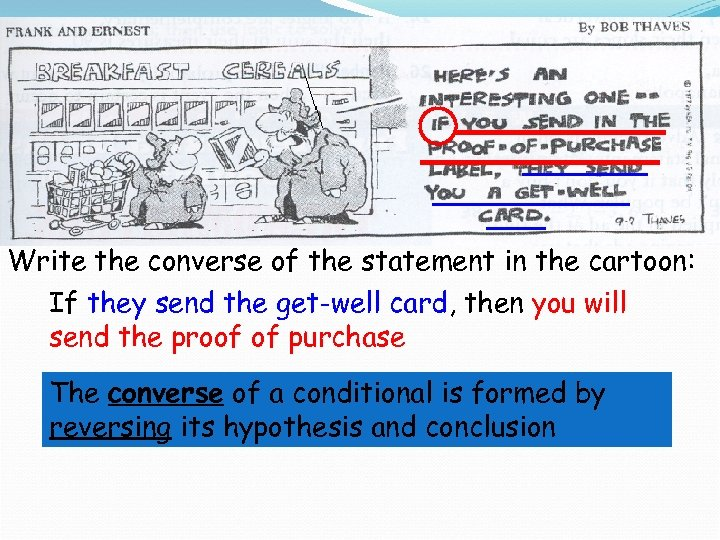Write the converse of the statement in the cartoon: If they send the get-well card, then you will send the proof of purchase The converse of a conditional is formed by reversing its hypothesis and conclusion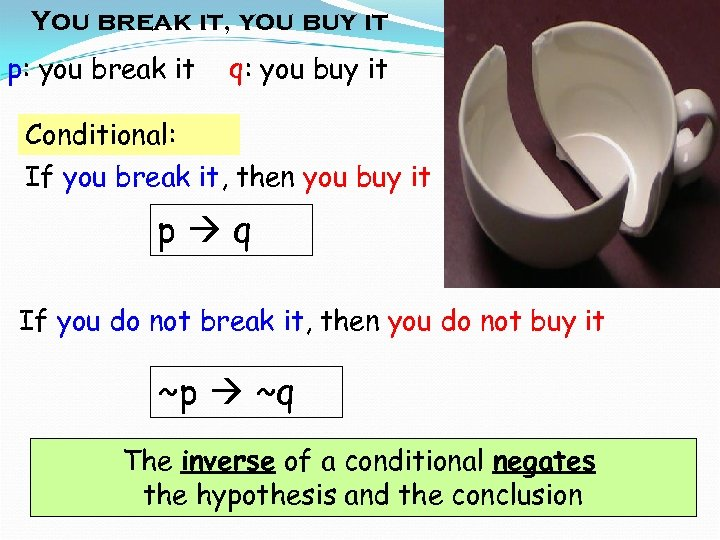You break it, you buy it p: you break it q: you buy it Conditional: If you break it, then you buy it p q If you do not break it, then you do not buy it ~p ~q The inverse of a conditional negates the hypothesis and the conclusion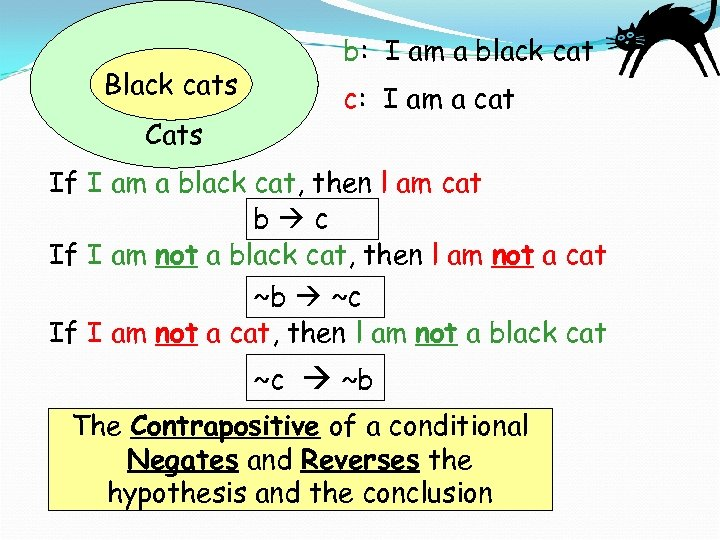Black cats Cats b: I am a black cat c: I am a cat If I am a black cat, then l am cat b c If I am not a black cat, then l am not a cat ~b ~c If I am not a cat, then l am not a black cat ~c ~b The Contrapositive of a conditional Negates and Reverses the hypothesis and the conclusion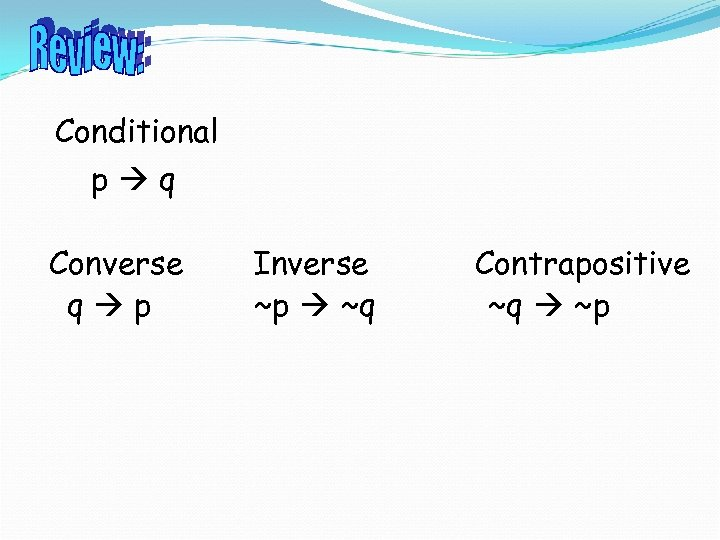Conditional p q Converse q p Inverse ~p ~q Contrapositive ~q ~p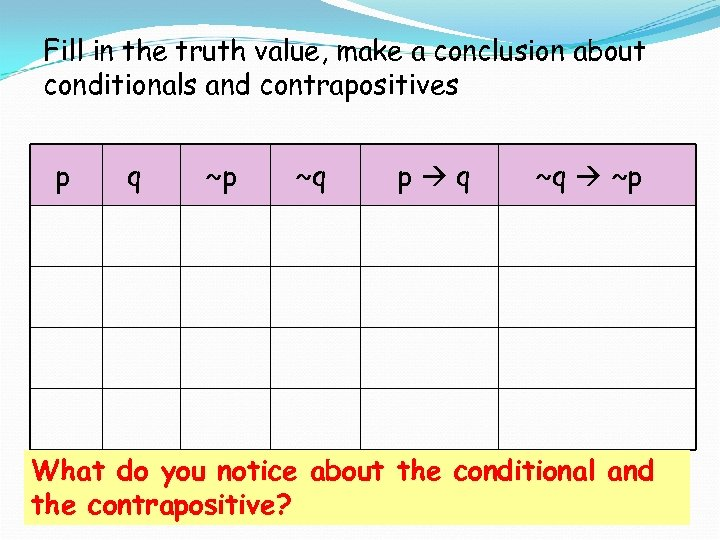Fill in the truth value, make a conclusion about conditionals and contrapositives p q ~p ~q p q ~q ~p What do you notice about the conditional and the contrapositive?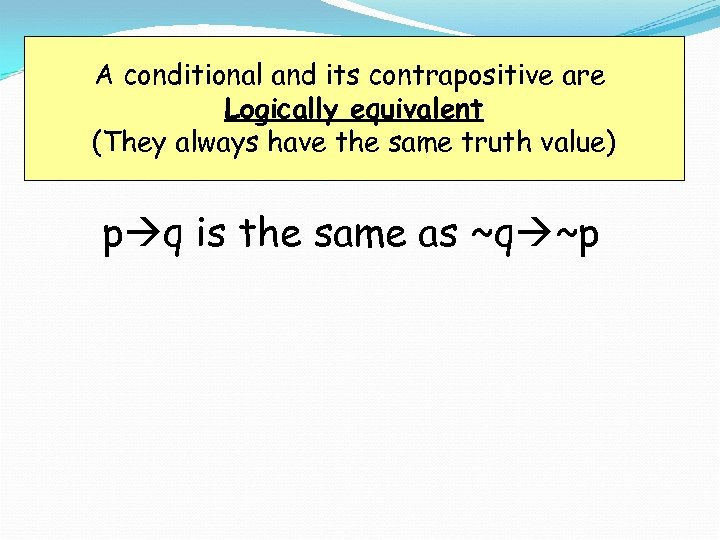A conditional and its contrapositive are Logically equivalent (They always have the same truth value) p q is the same as ~q ~p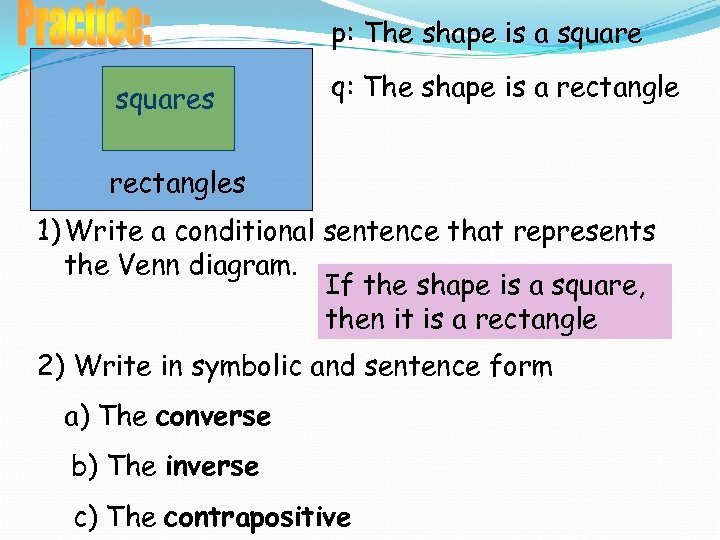p: The shape is a squares q: The shape is a rectangles 1) Write a conditional sentence that represents the Venn diagram. If the shape is a square, then it is a rectangle 2) Write in symbolic and sentence form a) The converse b) The inverse c) The contrapositive